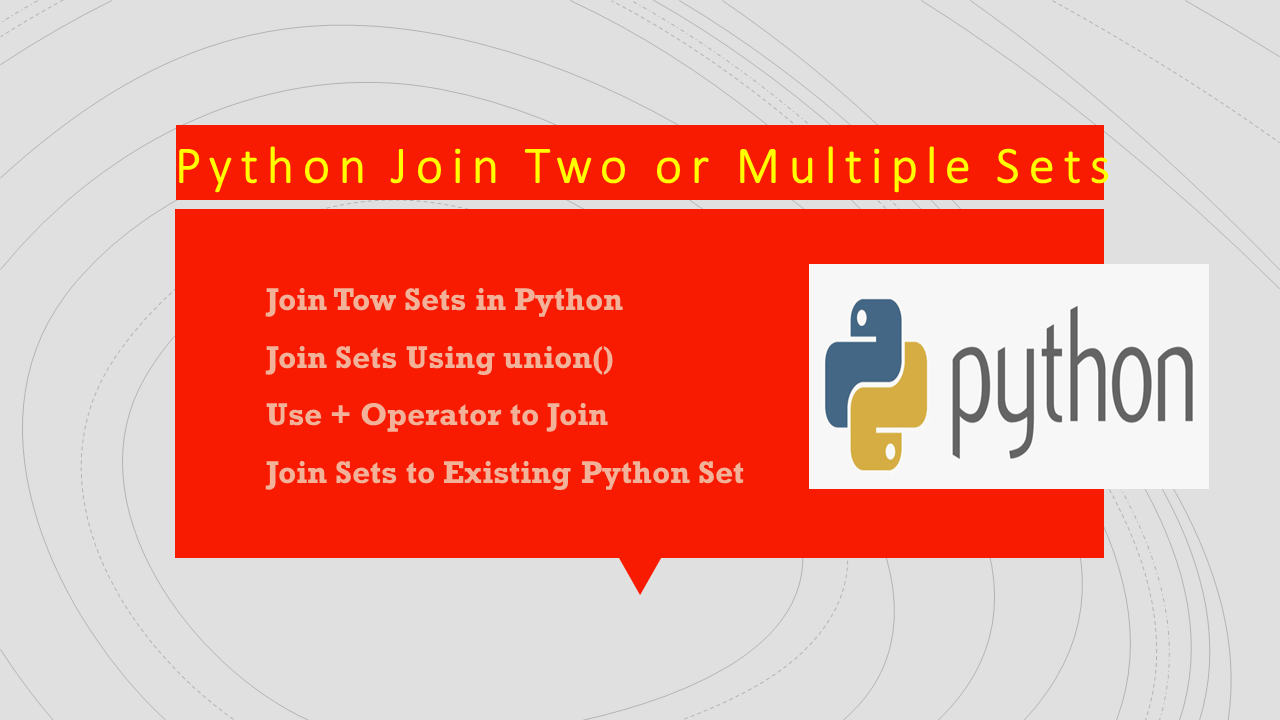# Python Join Two or Multiple Sets

How to join two or multiple sets in Python? There are several ways to join two or multiple sets. For example, you can either use the + operator, union() function, or union (|) operator. Besides these, you can also use the update() method in case you wanted to join to the existing set.

## 1. Quick Examples of Joining Two Sets

Following are quick examples of joining elements from two sets.

``````
# Quick Examples

myset = myset1 | myset2 | myset3

myset = myset1.union(myset2,myset3)

myset = set(list(myset1) + list(myset2))

myset.update(myset1,myset2)
``````

## 2. Join Tow Sets in Python

You can use the | operator to join two sets in Python. This combines the elements from both sets into a single set. By using this operator, you can also join multiple sets at a time. This would return a new set that contains all of the elements from `myset1` and `myset2`, without any duplicates.

``````
# Create sets
myset1 = {1,2,3,4,5,6}
myset2 = {7,8,9,1,4}

myset = myset1 | myset2
print(myset)

# Output:
# {1, 2, 3, 4, 5, 6, 7, 8, 9}
``````

Let’s also see another example with Strings. Here, I will join elements from three sets into a single set.

``````
# Create sets
set1 = {"one","two","three"}
set2 = {"four","five","six"}
set3 = {"one","four","nine"}

# Join sets with strings using |
myset = set1 | set2 | set3
print(myset)

# Output:
# {'one', 'six', 'two', 'five', 'four', 'nine', 'three'}
``````

## 3. Join Sets Using union()

The set.union() is the same as the | operator, which will join the values from two python sets. It can also be possible to join multiple sets at a time. Let’s use the same sets that were created above to join.

``````
# Join sets using union
myset = myset1.union(myset2)
print(myset)

# Output:
# {1, 2, 3, 4, 5, 6, 7, 8, 9}
``````

Similarly, you can join sets with strings.

``````
# Join sets with string using union()
myset = set1.union(set2,set3)
print(myset)

# Output:
# {'one', 'six', 'two', 'five', 'four', 'nine', 'three'}
``````

## 4. Use + Operator to Join

The ‘+’ operator is used to join two or multiple sets at a time in Python. We can’t use sets with the + operator hence, we need to convert the set to a list, join lists and convert the result from the list to a set.

Let’s create two sets with integer values and join them into a single set. To perform the conversion, I will use list() to convert the set to a list and set() to convert the resulting list to a set.

``````
# Join sets using +
myset = set(list(myset1) + list(myset2))
print(myset)

# Output:
# {1, 2, 3, 4, 5, 6, 7, 8, 9}
``````

## 5. Join Sets to Existing Python Set

All the above examples we have seen return a new set after joining values. To join sets the existing set use set.update() method.

``````
# Join elements to the existing set
myset1.update(myset2)
print(myset1)

set1.update(set2,set3)
print(set1)

# Output:
# {1, 2, 3, 4, 5, 6, 7, 8, 9}
# {'one', 'six', 'two', 'five', 'four', 'nine', 'three'}
``````

## 6. Conclusion

In this article, you have learned different ways to join two or multiple sets in Python by using the |, union(), update(), and + operators. Most of these returns a new set after joining elements and to join to an existing set use the update() function. Make sure that you need to convert the set to a list before applying the ‘+’ operator. Both ‘|’ and union() functionality is the same.

Happy Learning!!

### Naveen

I am a Data Engineer with 20+ years of experience in transforming data into actionable insights. Over the years, I have honed my expertise in designing, implementing, and maintaining data pipelines with frameworks like Apache Spark, PySpark, Pandas, R, Hive and Machine Learning. My journey in the field of data engineering has been a continuous learning, innovation, and a strong commitment to data integrity. I have started this SparkByExamples.com to share my experiences with the data as I come across. You can learn more about me at LinkedIn